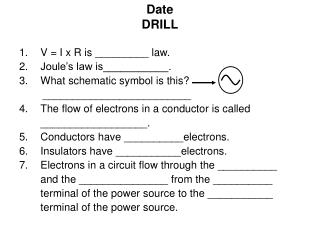Download PresentationDate DRILL

# Date DRILL - PowerPoint PPT PresentationDownload Presentation## Date DRILL

- - - - - - - - - - - - - - - - - - - - - - - - - - - E N D - - - - - - - - - - - - - - - - - - - - - - - - - - -
##### Presentation Transcript

1. DateDRILL • V = I x R is _________ law. • Joule’s law is___________. • What schematic symbol is this? _________________________ • The flow of electrons in a conductor is called __________________. • Conductors have __________electrons. • Insulators have ___________electrons. • Electrons in a circuit flow through the __________ and the _______________ from the __________ terminal of the power source to the ___________ terminal of the power source.

2. DateDRILL • V = I x R is __Ohm’s_______ law. • Joule’s law is _ P = V x I _____. • What schematic symbol is this? _AC Power Source_____________ • The flow of electrons in a conductor is called __Current_________. • Conductors have __free____electrons. • Insulators have __bound____electrons. • Electrons in a circuit flow through the Conductor and the ___Load________ from the negative__ terminal of the power source to the positive terminal of the power source.

3. U3e-L3 Resistance Calculations • Calculate the resistance in the following circuit: 100 Ω 100 Ω 100 Ω R3= R2= R1=

4. 1/RT = 1/R1 + 1/R2 + 1/R3 1/RT = 1/100 Ω + 1/100 Ω + 1/100 Ω 1/RT = .01 Ω + .01 Ω + .01 Ω 1/RT = .03 Ω 1 = .03 Ωx RT 1/.03 Ω= RT 33.333 Ω= RT

5. Draw a schematic for this circuit 25Ω 5Ω 4Ω 10Ω 120v 3Ω 2Ω

6. R6= 25Ω R3= 5Ω 120v R5= 4Ω R4= 10Ω R2= 2Ω R1= 3Ω

7. 1. Calculate the RT 2. Calculate the Current 3. Calculate the Power

8. Start by identifying • any parallel segments A R6= 25Ω R3= 5Ω 120v R5= 4Ω R4= 10Ω R2= 2Ω R1= 3Ω

9. 1a. Calculate parallel segments 1/RA = 1/R3 + 1/R4 + 1/R5 1/RA = 1/5 Ω + 1/10 Ω + 1/4 Ω 1/RA = .2 Ω + .1 Ω + .25 Ω 1/RA = .55 Ω 1 = RA x .55 Ω 1/.55 Ω= RA 1.818 Ω= RA

10. 1b. Calculate Total Resistance (RT) RT = R1 + R2 + RA + R6 RT = 3Ω+ 2Ω+ 1.818Ω+ 25Ω RT = 31.818Ω • Calculate Current V = I x R 120v = I x 3.818Ω 120v/3.818Ω= I 31.43 amps. = I

11. Calculate Power P = V x I P = 120 v x 31.43 amps. P = 3771.6 W

12. HOMEWORK 120v 10 amps. • Schematic • Resistance = ? • Power = ?

13. HOMEWORK • What type of power supply (AC or DC)? ___________ • 2. What is the voltage? _____________ • 3. What is the frequency? ___________ Hz. 100 v 50 v 0 v -50 v -100 v 0.3 sec 0.2 sec 0.1 sec